# Solution assignment 06 Logarithmic equations

### Assignment 6

Solve: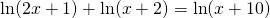### Solution

The domains of the subsequent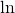functions are: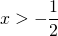and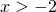and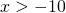thus:We rewite the equation as: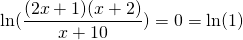and thus: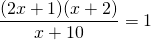Cross-multiplication yields: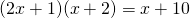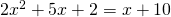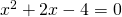The solutions of this equations are (applying the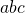-formula):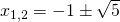The solution: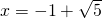lies in the domain and is valid. The other solution lies outside the domain and does not satisfy the equation.